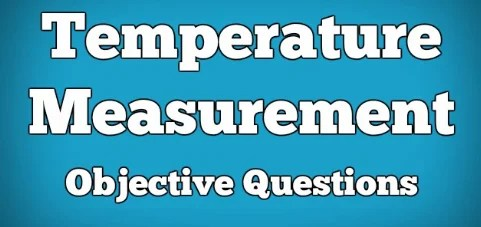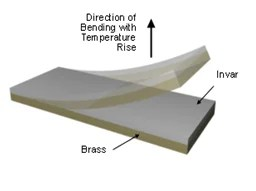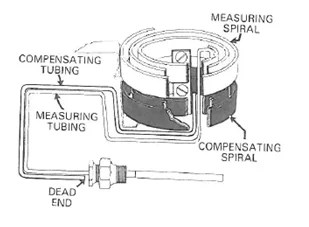# Temperature Measurement Instrumentation Questions Answers## Temperature Measurement Instrumentation Questions Answers

Q1. A thermocouple is installed in a tank to measure the temperature of the process liquid in the tank. What is the heat transfer mode from the liquid to the thermocouple tip ?

1. Conduction
2. Convection

Q2. Heat convection is  the transfer of heat energy through the action of a moving fluid. ( sate True or False )

1. TRUE                FALSE

Q3. One example of a liquid used in Class V filled system

1. Alcohol
2. Methyl chloride
3. Mercury
4. Silicon

Q4. 100°C is equal to

1. 373°F
2. 212°F
3. 32°F
4. -32°F

Q5. In a bimetallic thermometer, when the temperature element is exposed to heat, it will bent towards

1. The metal with lower coefficient of expansion
2. The metal with higher coefficient of expansion
3. Lower temperature side
4. Higher temperature sideQ6. An example for , negative temperature coefficient (NTC) device

1. Thermistor
2. RTD
3. Thermocouple
4. None of the above

Q7. Thermistors are not widely used in process measurements because

1. Its resistance increases with temperature
2. It has extremely high temperature coefficient
3. It is made of semiconductor material
4. Of its non linearity characteristic

Q8. Which of the following construction elements, RTDs and Thermocouples have in common ?

1. Sensing element
2. Sensor sheath

Q9. In an RTD, Lead wires are attached to the sensor sheath  ( True or false)

1. TRUE      B.FALSE

Q10.  Packing material used in the RTD probe around the sensor

1. Epoxy
2. Magnesium oxide
3. Teflon
4. Mercury

Q11. Resistance of a wire __________________ when the area of cross section of the wire decreases.

1. Decreases
2. Increases R=DENSITY  *  LENGTH  /  AREA
3. Remains same
4. Doubles

Q12. If the temperature coefficient of resistance of platinum is 0.00385Ω/Ω°C, how much is the resistance change for 1°C change in temperature ?

1. 1 Ω
2. 59 Ω
3. 385 Ω
4. 00385 Ω

Q13. What is the significance of 100  in Pt100 ?

1. 100 °C is the boiling point of water
2. At 100 °C, the resistance of platinum wire is 100 Ω
3. The atomic weight of platinum is 100
4. At 0 °C, the resistance of Pt100 is 100 Ω

Q14. To be useful as an RTD element, the metal or alloy must have the following characteristics ( choose all that apply)

1. Low melting point
2. A predictable relationship between temperature and resistance
3. Stability over the measured temperature range
4. A large, easily measurable change in resistance for a change in temp

Q15. Which RTD among the following have high accuracy, high repeatability, high resistance change per temperature and highly linear over its range

1. Platinum
2. Copper
3. Nickel

Q16. To compensate for lead wire effect in RTDs, the following configuration is used. (select all that apply)

1. Two wire RTD
2. Three wire RTD
3. Four wire RTD
4. None of the above.

Q17. If the lead wire resistance of an RTD is 4 Ω /lead , what is the error in the  temperature measurement of a 2 wire RTD ? ( Take α = 0.00385 Ω/Ω°C )

1. 21°C
2. 4 °C
3. 54 °C
4. 1 °C

Q18. Three wire RTD  is less accurate than 4 wire RTD. Why ?

1. Lead wire resistances of all the 3 leads may not be equal
2. Self heating effect
3. Only 3 wires are used
4. 3 wires introduce more error than 4 wires

Q19. Self heating effect is associated with

1. Thermocouples
2. Filled systems
3. Transmitters
4. RTDs

Q20. Which one of the following thermocouples is used for high temperature applications?

1. K- type
2. J-type
3. E-type
4. T-type

Q21. Seebeck effect is associated with

1. Thermistor
2. Bimetallic sensor
3. Thermocouple
4. RTD

Q22.  ____________ is the most common or preffered resistance metal to construct RTDs.

1. Platinum
2. Copper
3. Nickel
4. Gold

Q23. Which type of junctions in a thermocouple has the fastest response time?

1. Grounded
2. Ungrounded
3. Isolated
4. Unisolated

Q24. What sort of dual wire configuration enables a thermocouple to continue functioning even if one wire breaks ?

1. Grounded
2. Ungrounded
3. Isolated
4. Dual grounded

Q25. A voltage measurement at the cold junction of a T/C is proportional to the difference in temperature between the hot and cold junctions. ( True or False )

1. True
2. False

Q26. ______________ thermocouples are generally used in very low temperature application; can be used in moist atmosphere also.

1. Type J
2. Type K
3. Type E
4. Type T

Q27. Since iron wire is susceptible to oxidation, this thermocouple should only be used in dry atmosphere.

1. TypeJ
2. Type K
3. Type E
4. Type T

Q28. The hot junction temperature of a TypeJ T/C is 50 deg C . From the T/C table, the mV output for 50 deg C is 2.585 mV . If the reference junction temperature is 50 deg C, what will be the thermocouple output voltage ?

1. Zero
2. 585 mV
3. 50 mV
4. None of the above

Q29. If the measured output from a J type T/C is 13.998 mV and the reference junction is 25 deg C, what will be the actual measuring junction temperature  ?

1. 258
2. 303
3. 281
4. 345

Q30. How is the cold junction compensation performed in the controller or in the        transmitter ?

1. Subtracts the millivolt equivalent of the ambient temperature from the thermocouple output
2. Adds the millivolt equivalent of the ambient temperature to the thermocouple output
3. Subtracts the ambient temperature from the thermocouple output
4. Adds the ambient temperature to the thermocouple output

Q31. RTDs _________  highly  susceptible to noise and show _______________ linearity to temperature changes when compared to T/Cs.

1. are  ; higher
2. are ; lower
3. are not ; higher
4. are not ; lower

Q32. It is important to select correct extension wire for the thermocouples, because the use of an incorrect extension wire will cause damage to the thermocouple wires. (state True or false ).

1. TRUE
2. FALSE

Q33. Causes of incorrect thermocouple measurements

1. No shielding of T/C wires
2. Poor junction connections
3. Polarity reversal
4. All of the above

Q34. Which type of thermowell mounting is the weakest ?

1. Flanged
3.  Welded

Q35. The smaller the distance between the sensor sheath and thermowell, the  response.

_____________.

1. is slower
2. is Faster

Q36. Thermocouple functions at ___________ temperatures than RTDs

1. higher
2. lower
3. equal

Q37. Signal cable and power cable must not be housed in the same conduit. Why?

TO AVOID NOISE

Q38. In the following figure what is the purpose of providing the compensating tubing ?### 125 QUESTIONS ANSWERS TEMPERATURE INSTRUMENTATION ENGINEERING

paktechpoint.com

#### You may also like...

error: tected !!Content is pro